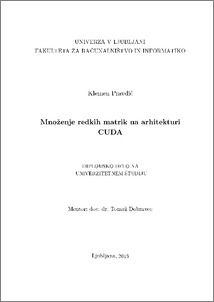# Sparse matrix multiplication on CUDA

Klemen Pravdič (2013) Sparse matrix multiplication on CUDA. EngD thesis.Preview
PDF

## Abstract

Sparse matrix multiplication is a common operation in linear algebra and an important element of other algorithms. Sparse matrix is a matrix populated primarily with zeros. This thesis presents two algorithms for sparse matrix multiplication, row-column algorithm and row-row (also known as row-wise) algorithm. It describes sequential implementation on CPU and parallel implementation on GPU for both algorithms. Algorithms were implemented in C programming language. For parallel implementation we used GPU with CUDA architecture. We described different formats of storage for sparse matrices (CSR, CSC, and COO) that are used in implementation of algorithms. For the purpose of understanding parallel implementations of algorithms CUDA architecture is described. Timings for all implementations were measured and compared against each other. For testing purposes we used sparse matrices from Matrix Market repository along with sparse matrices with different densities and dimensions that we generated ourselves. On GPU we stored product as both, a sparse and a dense matrix. We determined that row-row algorithm is faster than row-column algorithm and under certain conditions parallel implementation outperforms sequential implementation of a row-row algorithm. Performance of parallel row-row algorithm depends on density and dimensions of input matrices; for efficient performance input matrices with smaller dimensions should be denser. Row-row algorithm on CUDA performs better when groups of implicitly synchronized threads (warps) are used.

Item Type: Thesis (EngD thesis)
Keywords: Sparse matrix multiplication, sparse matrix, GPU processing, CUDA
Number of Pages: 51
Language of Content: Slovenian
Mentor / Comentors:
Name and SurnameIDFunction
doc. dr. Tomaž Dobravec258Mentor